Courses

# RD Sharma Solutions Ex-13.3, (Part -1), Linear Equation In Two Variables, Class 9, Maths Class 9 Notes | EduRev

## Class 9 : RD Sharma Solutions Ex-13.3, (Part -1), Linear Equation In Two Variables, Class 9, Maths Class 9 Notes | EduRev

The document RD Sharma Solutions Ex-13.3, (Part -1), Linear Equation In Two Variables, Class 9, Maths Class 9 Notes | EduRev is a part of the Class 9 Course RD Sharma Solutions for Class 9 Mathematics.
All you need of Class 9 at this link: Class 9

Q 1: Draw the graph of each of the following linear equations in two variables:

(i) x + y = 4
(ii) x – y = 2
(iii) -x + y = 6
(iv) y = 2x
(v) 3x + 5y = 15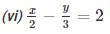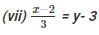Ans. (i) We are given, x + y = 4

We get, y = 4 – x,

Now, substituting x = 0 in y = 4 – x,

we get y = 4

Substituting x = 4 in y = 4 — x, we get y = 0

Thus, we have the following table exhibiting the abscissa and ordinates of points on the line represented by the given table

 X 0 4 Y 4 0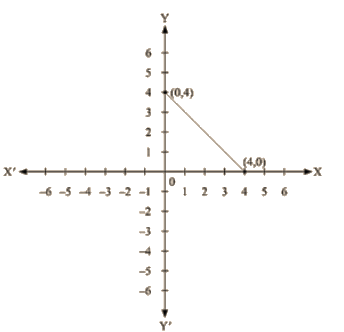(ii) We are given, x – y = 2

We get, y = x – 2

Now, substituting x = 0 in y= x – 2, we get y = – 2

Substituting x = 2 in y = x – 2, we get y = 0

Thus, we have the following table exhibiting the abscissa and ordinates of points on the line represented by the given equation

 X 0 2 Y -2 0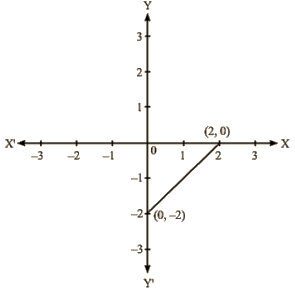(iii) We are given, – x + y = 6

We get, y = 6 + x

Now, substituting x = 0 in y = 6 + x,

We get y =6

Substituting x = -6 in y = 6+ x, we get y = 0

Thus, we have the following table exhibiting the abscissa and ordinates of points on the line represented by the given equation.

 X 0 -6 Y 6 0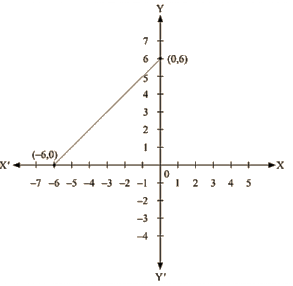(iv) We are given, y = 2x

Now, substituting x = 1 in y = 2x

We get y = 2

Substituting x = 3 in y = 2x

We get y = 6

Thus, we have the following table exhibiting the abscissa and ordinates of points on the line represented by the given equation

 X 1 3 Y 2 6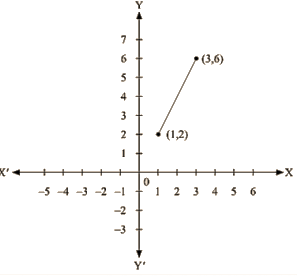(v) We are given, 3x + 5y = 15

We get, 15 – 3x = 5y

Now, substituting x = 0 in 5y = 15 – 3x,

We get; 5y = 15

y =3

Substituting x = 5 in 5y = 15 – 3x

we get 5 y = 0

Thus, we have the following table exhibiting the abscissa and ordinates of points on the line represented by the given equation

 X 0 5 Y 3 0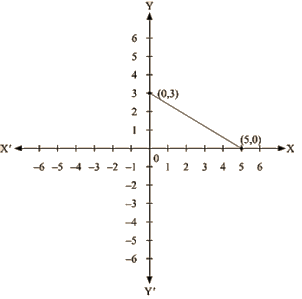(vi) we are given.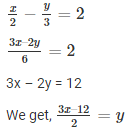Now, substituting x = 0 in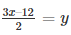We get y = -6

Substituting x = 4 in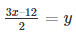We get y = 0

Thus, we have the following table exhibiting the abscissa and ordinates of points on the line represented by the given equation

 X 0 4 Y -6 0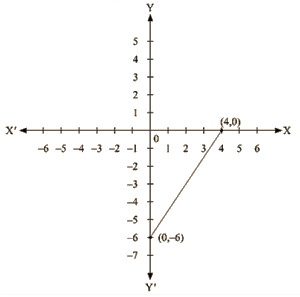(vii) We are given,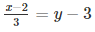We get, x-2 = 3(Y-3)

x – 2 = 3y – 9

x = 3y – 7

Now, substituting x = 5 in x = 3y – 7,

We get; y = 4

Substituting x = 8 in x  = 3y – 7 ,

We get; y = 5

Thus, we have the following table exhibiting the abscissa and ordinates of points on the line represented by the given equation

 X 5 8 Y 4 5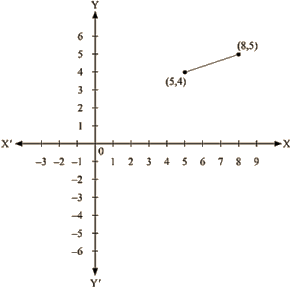(viii) We are given, 2y = – x +1

We get, 1 – x = 2Y

Now, substituting x =1 in1 – x = 2Y, we get

y = 0

Substituting x = 5 in 1 – x = 2Y , we get

y = – 2

Thus, we have the following table exhibiting the abscissa and ordinates of points on the line represented by the given equation

 X 1 5 Y 0 -2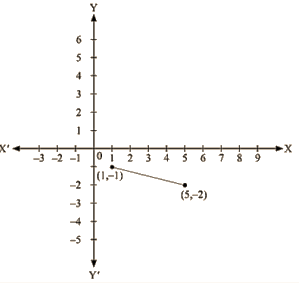Q 2: Give the equations of two lines passing through ( 3, 12). How many more such lines are there, and why?

Ans. We observe that x = 3 and y = 12 is the solution of the following equations

4x – y = 0 and 3x – y + 3 = 0

So, we get the equations of two lines passing through (3, 12) are, 4x – y = 0 and 3x – y + 3 = 0.

We know that passing through the given point infinitely many lines can be drawn.

So, there are infinitely many lines passing through (3, 12)

Q 3 : A three-wheeler scooter charges Rs 15 for first kilometer and Rs 8 each for every subsequent kilometer. For a distance of x km, an amount of Rs y is paid. Write the linear equation representing the above information.

Ans. Total fare of Rs y for covering the distance of x km is given by

y = 15 + 8(x – 1)

y = 15 + 8x – 8

y = 8x + 7

Where, Rs y is the total fare (x – 1) is taken as the cost of first kilometer is already given Rs 15 and 1 has to subtracted from the total distance travelled to deduct the cost of first Kilometer.

Q 4 : A lending library has a fixed charge for the first three days and an additional charge for each day thereafter. Aarushi paid Rs 27 for a book kept for seven days. If fixed charges are Rs x and per day charges are Rs y. Write the linear equation representing the above information.

Ans. Total charges of Rs 27 of which Rs x for first three days and Rs y per day for 4 more days is given by

x + y ( 7 – 3 ) = 27

x + 4y = 27

Here, (7 —3) is taken as the charges for the first three days are already given at Rs x and we have to find the charges for the remaining four days as the book is kept for the total of 7 days.

Q5: A number is 27 more than the number obtained by reversing its digits. lf its unit’s and ten’s digit are x and y respectively, write the linear equation representing the statement.

Ans. The number given to us is in the form of  ‘ yx ‘,

Where y represents the ten’s place of the number

And x represents the unit’s place of the number.

Now, the given number is 10y + x

Number obtained by reversing the digits of the number is 10x + y

It is given to us that the original number is 27 more than the number obtained by reversing its digits

So, 10y + x = 10x + y + 27

10y – y + x – 10x = 27

9y – 9x = 27

9 ( y – x ) = 27

y – x  = 27/9 = 3

x – y + 3 = 0

Q6: The Sum of a two digit number and the number obtained by reversing the order of its digits is 121. If units and tens digit of the number are x and y respectively, then write the linear equation representing the above statement.

Ans. The number given to us is in the form of ‘ yx’ ,

Where y represents the ten’s place of the number and x represents the units place of the number

Now, the given number is 10y + x

Number obtained by reversing the digits of the number is 10x+ y

It is given to us that the sum of these two numbers is 121

So, (10y + x)+ (10x + y) = 121

10y + y + x + 10x = 121

11y + 11x = 121

11 (y + x) = 121

x + y = 121/11 = 11

x + y = 11

Q7 : Plot the Points (3,5) and (-1,3) on a graph paper and verify that the straight line passing through the points, also passes through the point (1,4)

Ans.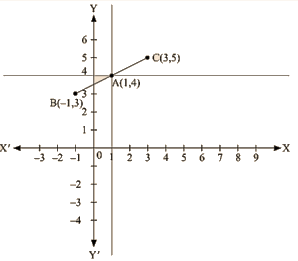By plotting the given points (3, 5) and (-1, 3) on a graph paper, we get the line BC.

We have already plotted the point A (1, 4) on the given plane by the intersecting lines.

Therefore, it is proved that the straight line passing through (3, 5) and (-1, 3) also passes through A (1, 4).

Offer running on EduRev: Apply code STAYHOME200 to get INR 200 off on our premium plan EduRev Infinity!

91 docs

,

,

,

,

,

,

,

,

,

,

,

,

,

,

,

,

,

,

,

,

,

,

,

,

,

,

,

,

,

,

,

,

,

;